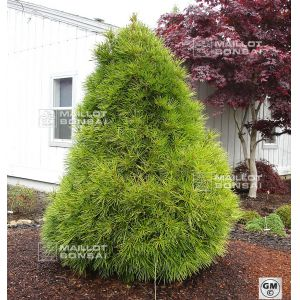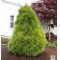##### The Japanese Bonsai specialist
Direct order Contact Help / Services Newsletter# Shelf to display

Pin parasol japonaisref. : 77

8,00

Available quantity : 2Order

###### Description

Sachet of + - 40 very fresl seeds of Japan are + - 2 grams of sciadopitys verticillata. Instructions for use include in the sachet.

Description: The Pine Sunshade of Japan in French is a scheming and unrecognized plant. He/it is from Japan and more especially of the island of Shikoku where he/it prospers in the nature. It is a big tree to the dense foliage that can reach about ten height meters. His/her/its foliage is composed of needles that confer him a very original aspect. Indeed, his/her/its needles are thick and of a length of 10 to 15 cm. The winter, his/her/its ramification and the color slightly bronze of his/her/its foliage is appreciated very by the Japanese amateurs.

Seedling: End March in terrine under cold frame. The seeds will be lying and not to the vertical.

The levee takes place at the end of eight to ten weeks, in a very variable proportion.

Earthy mixture: Thin sand, earth of garden, peels of small caliber pine, the finely sifted whole.

Plantation: To transplant individually in the beginning of the second year (while carving the radicelles that is very fine and fragile slightly) in a non enameled culture pot. The plantules will remain in their earth of seedling and will be put in greenhouse safe from frosts, until the beginning of the summer. To protect the direct sun also.

Fertilization: To fertilize (liquid manures) regularly and copiously. A complementary organic manure is recommended to assure to the plant a constant food.

Watering: The Sciadopitys especially fears the drought and the chalky water. To avoid the desséchement of the surface of the earthy mixture. To water copiously and regularly with the moderate water.

#seeds 6.5 #very 5.4 #sciadopitys 5.1 #verticillata 4.2 #foliage 3.7 #japan 3.5 #water 3.5 #that 3.4 #more 3.4 #will 3.4

Formule
(( ROUND((CHAR_LENGTH(b.article_nom)-CHAR_LENGTH(REPLACE(b.article_nom, 'very', '')))/LENGTH('very')) + ROUND((CHAR_LENGTH(b.article_description)-CHAR_LENGTH(REPLACE(b.article_description, 'very', '')))/LENGTH('very')) ) * 5.4) + (( ROUND((CHAR_LENGTH(b.article_nom)-CHAR_LENGTH(REPLACE(b.article_nom, 'sciadopitys', '')))/LENGTH('sciadopitys')) + ROUND((CHAR_LENGTH(b.article_description)-CHAR_LENGTH(REPLACE(b.article_description, 'sciadopitys', '')))/LENGTH('sciadopitys')) ) * 5.1) + (( ROUND((CHAR_LENGTH(b.article_nom)-CHAR_LENGTH(REPLACE(b.article_nom, 'seeds', '')))/LENGTH('seeds')) + ROUND((CHAR_LENGTH(b.article_description)-CHAR_LENGTH(REPLACE(b.article_description, 'seeds', '')))/LENGTH('seeds')) ) * 4.5) + (( ROUND((CHAR_LENGTH(b.article_nom)-CHAR_LENGTH(REPLACE(b.article_nom, 'verticillata', '')))/LENGTH('verticillata')) + ROUND((CHAR_LENGTH(b.article_description)-CHAR_LENGTH(REPLACE(b.article_description, 'verticillata', '')))/LENGTH('verticillata')) ) * 4.2) + (( ROUND((CHAR_LENGTH(b.article_nom)-CHAR_LENGTH(REPLACE(b.article_nom, 'foliage', '')))/LENGTH('foliage')) + ROUND((CHAR_LENGTH(b.article_description)-CHAR_LENGTH(REPLACE(b.article_description, 'foliage', '')))/LENGTH('foliage')) ) * 3.7) + (( ROUND((CHAR_LENGTH(b.article_nom)-CHAR_LENGTH(REPLACE(b.article_nom, 'japan', '')))/LENGTH('japan')) + ROUND((CHAR_LENGTH(b.article_description)-CHAR_LENGTH(REPLACE(b.article_description, 'japan', '')))/LENGTH('japan')) ) * 3.5) + (( ROUND((CHAR_LENGTH(b.article_nom)-CHAR_LENGTH(REPLACE(b.article_nom, 'water', '')))/LENGTH('water')) + ROUND((CHAR_LENGTH(b.article_description)-CHAR_LENGTH(REPLACE(b.article_description, 'water', '')))/LENGTH('water')) ) * 3.5) + (( ROUND((CHAR_LENGTH(b.article_nom)-CHAR_LENGTH(REPLACE(b.article_nom, 'will', '')))/LENGTH('will')) + ROUND((CHAR_LENGTH(b.article_description)-CHAR_LENGTH(REPLACE(b.article_description, 'will', '')))/LENGTH('will')) ) * 3.4) + (( ROUND((CHAR_LENGTH(b.article_nom)-CHAR_LENGTH(REPLACE(b.article_nom, 'that', '')))/LENGTH('that')) + ROUND((CHAR_LENGTH(b.article_description)-CHAR_LENGTH(REPLACE(b.article_description, 'that', '')))/LENGTH('that')) ) * 3.4) + (( ROUND((CHAR_LENGTH(b.article_nom)-CHAR_LENGTH(REPLACE(b.article_nom, 'especially', '')))/LENGTH('especially')) + ROUND((CHAR_LENGTH(b.article_description)-CHAR_LENGTH(REPLACE(b.article_description, 'especially', '')))/LENGTH('especially')) ) * 3)

## Secure payment## Delivery

Our logistic partners :